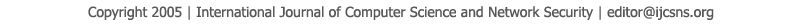To search, Click below search items.All Published Papers Search Service
 Title Author Year Keyword
 Title Positive solutions of fuzzy fractional Volterra integro-differential equationswith the Fuzzy Caputo Fractional Derivative using the Jacobi polynomials operational matrix Author M.R.Nourizadeh, T. Allahviranloo, N. Mikaeilvand Citation Vol. 18  No. 1  pp. 241-252 Abstract In this paper study,we introduce fuzzy fractional Volterraintegro- differentional equations (FFVIDEs) under Caputo generaliuzed Hukuhara differentiability.There are many applications of the G- derivative ,but we give positive solutions for (FFVIDEs)under Riemann -Liouville gH-differentiability using theJacobi polynomials operational matrix. Weintroduce Riemann -Liouville gH-differentiability as a direct generalization of the concept of fuzzy Caputo differentiability in a deterministic sense for a fuzzy context.Wepropose a computational method based on the tau method with Jacobi polynomials for FFVIDEs of order 0<β<1. The efficiency and applicability of the proposed method are demonstrated by several test examples. Finally,we give some illustrative examples. Keywords Caputo H-differentiability, fuzzy-valued function, fuzzy fractional Volterra integro-differential equations, Jacobi polynomial operational matrix, Positive solution URL http://paper.ijcsns.org/07_book/201801/20180131.pdf